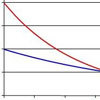#### You may also like### PDF

Given a probability density function find the mean, median and mode of the distribution.### Scale Invariance

By exploring the concept of scale invariance, find the probability that a random piece of real data begins with a 1.### Into the Exponential Distribution

Get into the exponential distribution through an exploration of its pdf.

# Into the Normal Distribution

##### Age 16 to 18Challenge Level

You might like to recall that the pdf of an $N(\mu, \sigma^2)$ random variable is
$$f(x) =\frac{1}{\sqrt{2\sigma ^2}}e^{-\frac{(x-\mu)^2}{2\sigma^2}}$$
and that the probability of a value falling between $a$ and $b$ is
$$P(a< x< b) = \int^b_a f(y)dy$$
Don't forget that the integral is the area under the curve between two points.

Don't forget that you can look up the cumulative probabilities for a normal distribution using tables.